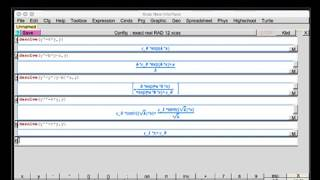#### Næste

Maple intro (Formler og ligninger)
skoleflix · 3 Visninger

# Two free alternatives to Maple & Mathematica

2 Visninger

Two free alternatives to Maple &
mathematical software Wolfram Mathematica
are

Xcas

https://www-fourier.ujf-grenob....le.fr/~parisse/giac.

(for Linux, Microsoft Windows and Apple macOS 32 bit)

and

ExpressionsinBar

http://www.alelvisoftware.com/....Expressions/Expressi

(for macOS 64 bit) in Mac App Store

------------------------------------------------------
Some commands for both free apps:

solve equation: solve(equation,x)

calculate derivative: diff(function,x)

calculate antiderivative: int(function,x)

solve differential equation: desolve(y'=k*y,y)

-----------------------------------------------------
Xcas solves differential equations

ExpressionsinBar can solve differential equation

free alternative to Maplesoft & Wolfram Mathematica
mathematics software mathematical program

The features of these free apps should be built in

Vis mere
0 Kommentarer